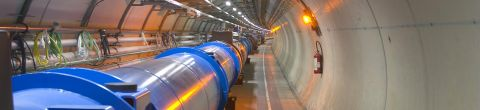Particle Physics 3: Supersymmetry and Grand UnificationSpring, 2010

In this set of lectures Professor Susskind continues his particle physics theme, moving on to supersymmetry, which describes the relationship between fermions and bosons.  He connects this to the concept of vacuum energy, the energy density associated with “empty” space.    Professor Susskind finishes with supersymmetry breaking and grand unified field theories, which predict proton decay.  (Image credit: Atlas Experiment at the Large Hadron Collider)

Lectures in this Course

1. Renormalization concepts, and dimensional analysis

In the first lecture of the series Professor Susskind introduces the concept of renormalization, which allows elimination of as yet unknown physics at very tiny scales or high energies from our calculations of physics at accessible scales. He also... [more]
2. Fermions and bosons

Professor Susskind starts with the topic of rotations, showing that particles under rotation by 2π either return to their initial state (wavefunction) or to their initial wavefunction multiplied by a phase of -1. The first case corresponds to... [more]
3. Propagators and renormalization of mass

This lecture reviews the propagator and connects its form to dimensional analysis.   Then loop propagators are used to introduce mass renormalization.
4. Symmetry and Grassmann numbers

Professor Susskind reviews the mathematical concepts of symmetry in preparation for the development of supersymmetry.   Then he introduces the mathematical concept of Grassmann numbers, which are used in the description of fermionic fields.
5. A first supersymmetric model

The lecture finishes the development of Grassmann numbers with the introduction of integration and differentiation.   With these tools in place Prof Susskind describes a simplified supersymmetric model.
6. Supersymmetry building blocks

Professor Susskind introduces superfields and integration with Grassmann variables.
7. Lagrangians that preserve supersymmetry

This lecture develops the notion of a Supercharge, Q+ representing the transformation from a fermion to a boson and Q the transformation from a boson to a fermion.
8. Generalizing supersymmetry to 3+1 spacetime, and QFT

In this lecture, Professor Susskind generalizes supersymmetry to 3 space and 1 time dimension.
9. Supersymmetry breaking and an introduction to grand unified theories

In the first half of the lecture, Professor Susskind makes an analogy between breaking supersymmetry and breaking the symmetry of a ferromagnet.   In the second half of the lecture, Professor Susskind introduces GUTs as corresponding to the group SU... [more]
10. GUTs, the SU(5) representation, proton decay

The final lecture focuses on grand unified theories, and how their group structure connects to fermions (neutrinos, leptons and quarks) and the gauge bosons.